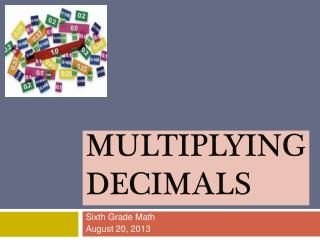DownloadDownload PresentationMultiplying Decimals

# Multiplying Decimals

Télécharger la présentation## Multiplying Decimals

- - - - - - - - - - - - - - - - - - - - - - - - - - - E N D - - - - - - - - - - - - - - - - - - - - - - - - - - -
##### Presentation Transcript

1. Multiplying Decimals Sixth Grade Math August 20, 2013

2. Essential Question: How do I multiply decimals using the standard algorithm for the operation?

3. Step 1: Write the longer number on top when setting up the problem. Line up the last digits of both numbers. Step 2: Multiply the numbers ignoring the decimal point(s). Step 3: Count the total number of digits to the right of each decimal point. Step 4: Start at the end of the product and move the decimals point to the left as many places as you counted in step 3.

4. Example 1 • Multiply 0.3 and 0.5 • Set up the problem 0.3 • x 0.5 1 1 Multiply .15 2 Count 15 Put the decimal point into the product

5. Notice: • 3 tenths of the columns are shaded • 5 tenths of the rows are shaded • The number of shaded squares that overlap are 15 out the 100 squares 50 squares 30 squares 15squares Let’s See It Another Way

6. 0.3 x 0.8 0.24 24 squares overlapping 30 squares 80 squares Example 2

7. Example 3 • Find the product of 9.683 and 6.1 9.683 x 6.1 9683 There are 4 digits behind the decimal point in the product. + 580980 590663 59.0663